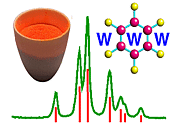Crystallographic Point Groups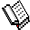Course Material Index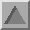Section Index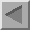Previous Page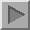Next Page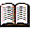The majority of the table is reference material.

Crystallographic Point Groups

The simplest crystallographic point groups are 1, 2, 3, 4, and 6 all of which possess a single rotation axis only. Likewise the rotary-inversion axes are the basis for the point groups -1, m, -3, -4, and -6. The remaining 22 crystallographic point groups result from the combination of the previous 10 point groups. With the exception of point groups belonging to the cubic crystal system, only twofold rotation axes and/or mirror planes can be taken together with other rotation or rotary-inversion axes: Twofold axes can be combined perpendicular to other axes (e.g. point-group 422) while mirror planes can act either perpendicular (e.g. 2/m) or parallel to another axis (mm2). A slash ("/") character before the symbol m indicates a mirror plane perpendicular to the main axis of rotation.

The crystallographic point groups may be classified according to the crystal system with which they are associated. Thus the point groups of the trigonal crystal system all possess a threefold axis, while those of the tetragonal and hexagonal crystal systems possess a fourfold and sixfold axis, respectively. The cubic point groups all have multiple threefold axes (see below). The orthorhombic point groups have twofold symmetry (either 2 or m with respect to each of the X-, Y-, Z- directions of an orthogonal axis system, while the monoclinic point groups are limited to twofold symmetry with respect to a single axis direction. Finally, the triclinic point groups can only have an axis of order 1.

The cubic point groups are all characterised by the four threefold rotation axes which act along the body diagonals of a cube. This is indicated by the digit "3" in the cubic point-group symbols. In addition, the cubic point groups all contain at least three mutually perpendicular twofold rotation axes.

Crystal System 32 Crystallographic Point Groups
Triclinic 1 -1
Monoclinic 2 m 2/m
Orthorhombic 222 mm2 mmm
Tetragonal 4 -4 4/m 422 4mm -42m 4/mmm
Trigonal 3 -3 32 3m -3m
Hexagonal 6 -6 6/m 622 6mm -62m 6/mmm
Cubic 23 m-3 432 -43m m-3m

Of the 32 crystallographic point groups, those highlighted in magenta possess a centre of inversion and are called centrosymmetric, while those highlighted in red possess only rotation axes and are termed enantiomorphic. A third type, highlighted in bold type, are referred to as polar. The properties of these different types of point groups are explained in more detail in the subsequent sections.Note: this additional material is given for completeness.

The ordering of the symmetry elements that compose the point-group symbols depends on the crystal system to which they are related. Thus, for the orthorhombic crystal system, the order of the components of the point-group symbol relates to the symmetry for the X,Y,Z directions, respectively. For 222 and mmm the order is immaterial, but for mm2 it is purely a convention that the rotation axis is chosen along Z. For the trigonal, tetragonal, and hexagonal point groups, which possess rotation axes of order 3, 4, and 6, respectively, the convention is to put the high symmetry rotation axis first in the symbol. The second component of the symbol refers to the symmetry with respect to the X axis (and also Y axis assuming Y is chosen in the crystallographic sense for the trigonal/hexagonal case), and again the highest-order rotation axis is chosen. Following these simple rules, one obtains point-group symbols such as -42m and -62m, but not -4m2 and -6m2.

However, the above rules only apply in the absence of a set of references axes. With reference to a crystallographic unit cell, it may happen that the twofold rotation axes, for example, in -42m refers to the diagonal direction between the a and b axes, but neither to a or b, while the normal for the mirror plane may refer to the a and b axes. Under these conditions, it would be correct to use the point-group symbol -4m2. The use of -4m2 is illustrated in a couple of point-group figures. (Point group figures are discussed at length in the following page). Note that there is no difference in the properties of point groups -42m and -4m2 other than one of axes labelling, but there is a difference when it comes to space groups (which form the subject of the next section on symmetry).

By contrast m-3 and -3m are quite different point groups. The cubic crystal system is always characterised by four threefold rotation axes. In addition, the higher-symmetry cubic point groups possess three fourfold rotation (or rotary-inversion) axes. If the highest-symmetry axis is put first, then the logical order is, for example, 432 or -43m, with the symbol for the threefold axis in second place. For consistency, all of the cubic point-group symbols (and space-group symbols too) have the characteristic 3 (or -3) as the second element of the point-group symbol. In addition, this avoids confusion with the trigonal system where only a single 3 (or -3) axis is present. Thus, for cubic systems the first component of the point-group symbol refers to symmetry with respect to the X, Y, and Z directions, the second refers to symmetry with respect to the body-diagonal of a cube, and the final component (if present) refers to symmetry with respect to the face-diagonal directions.Course Material IndexSection IndexPrevious PageNext Page
 © Copyright 1995-2006.  Birkbeck College, University of London. Author(s): Jeremy Karl Cockcroft